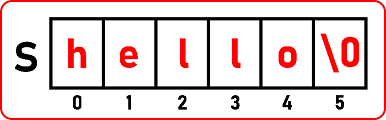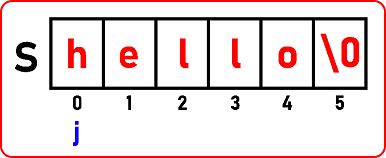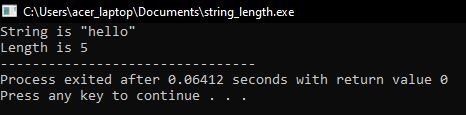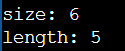# How to Find Length of a String in C

## How to Find Length of a String in C Language with Examples

In this article, I will discuss How to Find the Length of a String in C Language with Examples. Please read our previous article discussing Predefined String Functions in C Language with Examples.

##### How to Find the Length of a String in C Language?

Finding the length of a string in C is a common operation and can be done using the strlen() function provided by the standard C library. This function is defined in the <string.h> header file. But here, first, I will show you how to find the length of a string without using the strlen() function, i.e., we will write the custom logic to find the length of a string, and then we will see how we can use the built-in strlen() function to find the length of a given string. Please consider the following string.We want to find the length of the above string, which means the number of characters in a string. So, we must count all the characters until we reach ‘\0’. Here, the length of the string is 5, which means there are 5 characters in the array.

There are 5 alphabets, as the indices are starting from 0 onwards. So, at 5, we have ‘\0’. We know that the array size is 6, but the string size is 5, as we are not counting the null character (‘\0’). The size of an array can be anything, which can be bigger than the size of the string. With the help of ‘\0’, we can find the length of the string. So, the procedure is very simple. We have to scan this array of characters until we reach ‘\0’.So, for that, we can take our individual pointer j and go on looking for ‘\0’. So below is the full program for finding the length of a string in C Language

##### Program to Find the Length of a String in C Language:

In the below example, we are using a for loop to find the length of a string.

```#include <stdio.h>
#include <stdlib.h>
int main ()
{
char *S = "hello";
int j;
for (j = 0; S[j] != '\0'; j++)
{
// no code here
}
printf ("String is \"%s\"\n", S);
printf ("Length is %d", j);
return 0;
}```
###### Output:##### How to Find Length of a String Using Built-in strlen() String Function?

Using this strlen() predefined function, we can find the length of a given string in C Language. The strlen() function requires 1 argument of type (const char*) and returns an int type. When we are working with strlen() from the given address up to \0, the entire character count value will return. For a better understanding, please look at the following example:

```#include<stdio.h>
#include<string.h>
#include<conio.h>
int main()
{
char str[] = "hello";
int s,l;
s=sizeof(str);
l=strlen(str);
printf("\nsize: %d",s);
printf("\nlength: %d",l);
getch();
return 0;
}
```
###### Output: